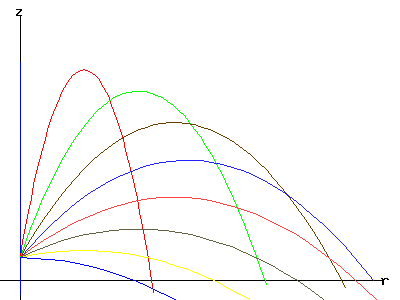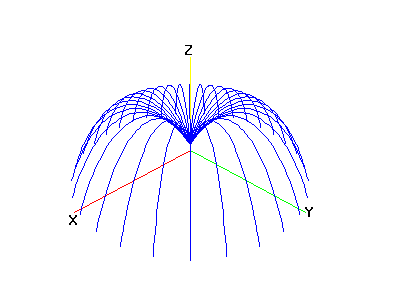Egwald Mathematics: Geometry - Trajectory of a Projectiles

by

Elmer G. Wiens

Egwald's popular web pages are provided without cost to users.
Please show your support by joining Egwald Web Services as a Facebook Fan:Follow Elmer Wiens on Twitter:A projectile is fired from a gun that is mounted on a cliff above the sea. What is the range of the gun? At what angle should the gun be fired to hit a ship within its range?
z0 = height of the gun above the x-y plane.
v0 = muzzle velocity = initial velocity of the projectile.
alpha = the angle between the horizontal (the x-y plane) and the muzzle of the gun.
z(t) = the height of the projectile t seconds after being fired.
r(t) = the distance of the projectile from the gun after t seconds.
g = pull of gravity.
Then the parametric equations of motion of (r(t), z(t)) are:
 r(t) = v0 * cos(alpha) * t z(t) = -1/2 * g * t^2 + v0 * sin(alpha) * t + z0
The trajectory of the
particle for varying angles alpha.The trajectory of the
particle in varying directions.Angle of elevation of the gun muzzle: alpha [0, 1.572] 1.0471975511966

Change the angle alpha (in radians, try .75) and click "Submit" to view the trajectories of the particle at the new angle.Copyright © Elmer G. Wiens:   EgwaldWeb ServicesAll Rights Reserved.    Inquiries